CHIRAG GOYAL — July 9, 2021

This article was published as a part of the Data Science Blogathon

## Introduction

If you start to learn deep learning, the first thing you will be exposed to is the concepts of Linear Algebra which give you better intuition on how algorithms really work under the hood, which enables you to make better decisions. In Deep Learning, a feed-forward neural network is a most simple and highly useful network. Under the hood, the feed-forward neural network is just a composite function, that multiplies some matrices and vectors together.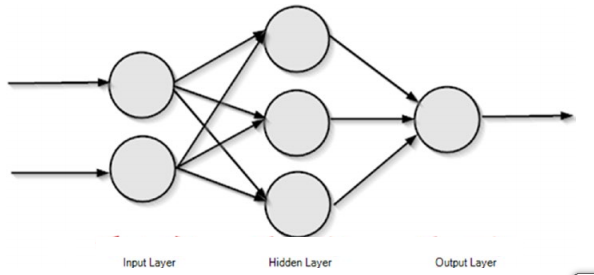Image Source:

It is not that vectors and matrices are the only way to do these operations but they become highly efficient if you do so. The core data structures behind Deep-Learning includes

• Scalars,
• Vectors,
• Matrices, and
• Tensors.

Matrix operations are used in the description of many Deep learning algorithms.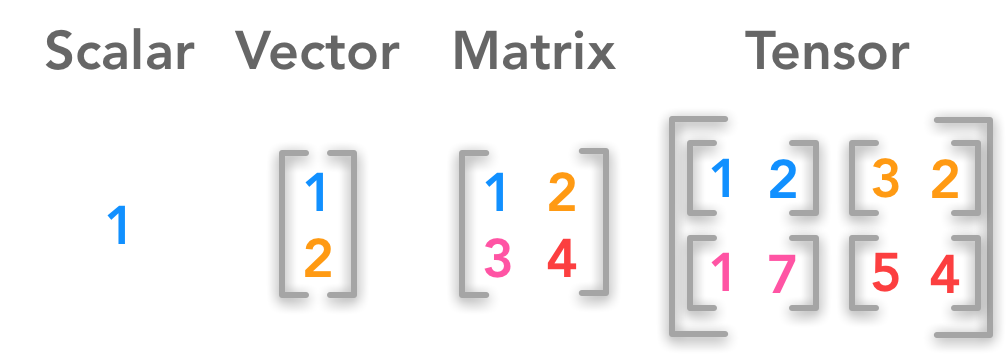Image Source:

So if you really want to be a professional in the field of Deep Learning, then you cannot escape mastering some of these concepts. So, In this article, we will discuss important linear algebra matrix operations that are used in the description of deep learning methods.

The topics which we will be discussing in this article are as follows:

• What are Matrices?
• How to add and subtract different matrices?
• How to find the shape and size of a given matrix?
• How to convert a dense matrix to a sparse matrix?
• How to find the transpose of a matrix?
• How to find the mean, variance, and standard deviation of a matrix?
• How to find the trace of a matrix?
• How to extract the minimum and maximum elements from a matrix?
• How to find the determinant of a matrix?
• How to multiply the given matrices?
• How to apply the particular operation to each element of a matrix?
• How to find the inverse of a matrix?
• How to reshape the matrix to a different size?

## What are Matrices?

Matrices are rectangular arrays consisting of numbers and can be seen as 2nd-order tensors. If m and n are positive integers, that is m, n ∈ ℕ then the m×n matrix contains m*n numbers of elements, with m number of rows and n number of columns.

The pictorial representation of an m×n matrix is shown below: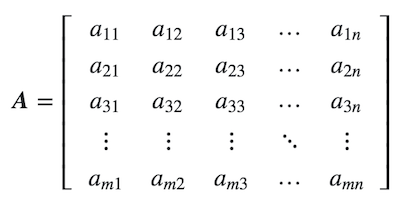Image Source:

Sometimes, instead of describing the full matrix components, we use the following abbreviation of a matrix: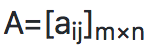### For Example-

In this example, with the help of the numpy library, we will create a matrix. And also check the dimension of the formed matrix.

```import numpy as np
matrix = np.array([[45,34],[67,58]])
# Create a matrix
print("The original matrix is given by n", matrix)
# Check the dimension of the matrix
print("The dimension of the given matrix is", matrix.ndim)```

Output:

```The original matrix is given by
[[45 34]
[67 58]]
The dimension of the given matrix is 2```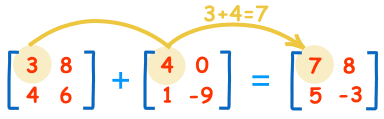Image Source:

In this section, we will be doing matrices addition and subtraction using the methods add and subtract. These methods take two arguments and return the sum and difference of those matrices respectively. If the shape of the matrices is not the same it throws an error saying, the addition or subtraction not possible.

```matrix_1 = np.array([[45,34],[67,58]])
matrix_2 = np.array([[35,24],[57,48]])
print("The result after adding matrix 1 and matrix 2 is given by n" , np.add(matrix_1, matrix_2))
# Subtract one matrix from the other matrices
print("The result after subtracting matrix 1 from matrix 2 is given by n" , np.subtract(matrix_1, matrix_2))
print("The result after subtracting matrix 2 from matrix 1 is given by n" , np.subtract(matrix_2, matrix_1))```

Output:

```The result after adding matrix 1 and matrix 2 is given by
[[ 80  58]
[124 106]]
The result after subtracting matrix 1 from matrix 2 is given by
[[10 10]
[10 10]]
The result after subtracting matrix 2 from matrix 1 is given by
[[-10 -10]
[-10 -10]]```

## Shape and Size of a Matrix

In this section, we will be finding shape i.e, the number of rows and columns in the given matrix and size i.e, number of elements in the matrix of a given matrix.

```matrix = np.array([[45,34,75],[67,58,89]])
# Finding number of rows and columns in the matrix
print("The number of rows and columns in the given matrix are " + str(matrix.shape) + " and " + str(matrix.shape) + " respectively")
# Number of elements in the matrix
print("The size of the given matrix is" , matrix.size)```

Output:

```The number of rows and columns in the given matrix are 2 and 3 respectively
The size of the given matrix is 6```

## Converting a given Dense matrix to a Sparse Matrix

Let’s first understand what exactly it means by Sparse and Dense Matrix.

A sparse matrix is a matrix that consists of mostly zero values. And Sparse matrices are different from matrices with mostly non-zero values, which are Known as dense matrices.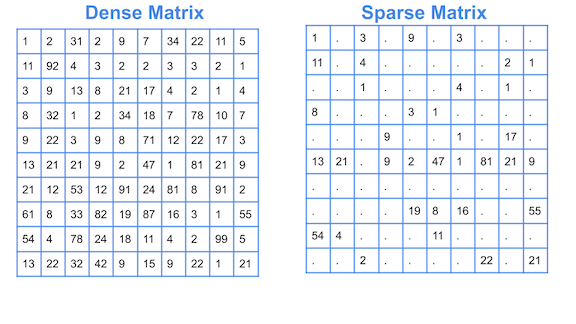Image Source:

```from scipy import sparse
# Create a Dense Matrix
dense_matrix = np.array([[0,0],[0,17],[78,0]])
# Convert Dense matrix to Sparse matrix
sparse_matrix = sparse.csr_matrix(dense_matrix)
print("The sparse matrix corresponding to a given dense matrix is given by n" , sparse_matrix)```

Output:

```The sparse matrix corresponding to a given dense matrix is given by
(1, 1)	17
(2, 0)	78```

## Matrix Transpose

In Matrix Transpose, we can convert a row vector to a column vector and vice versa i.e, row becomes columns and columns becomes rows.

If we have matrix A = [aij]mxn, then the transpose of this matrix is A= [aji]n×m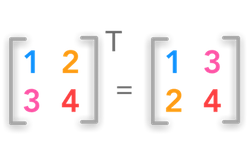Image Source:

```import numpy as np
matrix = np.array([[45,34],[67,58]])
print("The original matrix is given by n" , matrix)
print("The transpose matrix of the given matrix is n" , matrix.T)```

Output:

```The original matrix is given by
[[45 34]
[67 58]]
The transpose matrix of the given matrix is
[[45 67]
[34 58]]```

## Mean, Variance, and Standard Deviation of a Matrix

In this section, we will try to find some statistical things related to a matrix. Here we calculate the mean, variance, and standard deviation of the matrix using the numpy functions.

```import numpy as np
matrix = np.array([[45,34],[67,58], [23,89]])
# Finding the mean of a matrix elements
print("The mean of the elements of a matrix is equal to", np.mean(matrix))
# Finding the Variance of a matrix elements
print("The variance of the elements of a matrix is equal to", np.var(matrix))
# Finding the Standard Deviation of a matrix elements
print("The standard deviation of the elements of a matrix is equal to", np.std(matrix))
print("The standard deviation of the elements of a matrix is equal to", np.sqrt(np.var(matrix)))```

Output:

```The mean of the elements of a matrix is equal to 52.666666666666664
The variance of the elements of a matrix is equal to 473.5555555555555
The standard deviation of the elements of a matrix is equal to 21.761331658599286
The standard deviation of the elements of a matrix is equal to 21.761331658599286```

## Trace of a MatrixImage Source:

In this section, we will try to find the trace of a matrix i.e, the sum of all the diagonal elements present in a given matrix.

```import numpy as np
matrix = np.array([[1,2,3],[4,5,6], [7,8,9]])
# Get the diagonal elements of a matrix
print("The diagonal elements of a given matrix are n", matrix.diagonal())
# Finding the trace of the matrix
print("The trace of a given matrix is equal to", matrix.diagonal().sum())```

Output:

```The diagonal elements of a given matrix are
[1 5 9]
The trace of a given matrix is equal to 15```

## Finding minimum and maximum elements from a Matrix

In this section, we will try to find the minimum and maximum elements of a matrix i.e, the element with the highest and lowest value among all the elements.

```import numpy as np
matrix = np.array([[1,2,3],[4,5,6], [7,8,9]])
# Find the minimum element of the matrix
print("The minimum element in a given matrix is", np.min(matrix))
# Find the maximum element of the matrix
print("The maximum element in a given matrix is", np.max(matrix))```

Output:

```The minimum element in a given matrix is 1
The maximum element in a given matrix is 9```

## Determinant of a MatrixImage Source:

In this section, we will try to find the determinant of a matrix. Here to calculate the determinant, we use the linear algebra module present in the Numpy package.

```import numpy as np
matrix = np.array([[1,2,4],[3,4,6], [7,8,5]])
# Find the determinant of the matrix
print("The determinant of the given matrix is equal to", np.linalg.det(matrix))```

Output:

`The determinant of the given matrix is equal to 9.999999999999993`

## Matrix Multiplication

A matrix of shape (m x n) and B matrix of shape (n x p) multiplied gives C of shape (m x p). Remember while multiplying the matrices is that the number of columns in the first matrix is the same as the number of rows in the second matrix to perform multiplication without error.Image Source:

In this section, we will try to find the multiplication of two matrices.

```import numpy as np
matrix_1 = np.array([[45,34],[67,58]])
matrix_2 = np.array([[35,24],[57,48]])
print("The matrix multiplication of given two matrices is given by n", np.matmul(matrix_1, matrix_2))```

Output:

```The matrix multiplication of given two matrices is given by
[[3513 2712]
[5651 4392]]```

## Element wise operations using an inline function (Lambda)

In this example, we will try to add a certain value to each of the elements of a matrix.

```import numpy as np
matrix = np.array([[1,2,4],[3,4,6], [7,8,5]])
print("The matrix after adding 5 to all its elements is n", add_5_vec(matrix))```

Output:

```The matrix after adding 5 to all its elements is
[[ 6  7  9]
[ 8  9 11]
[12 13 10]]```

## Inverse of a Matrix

In this section, we will try to find the inverse of a matrix.

```import numpy as np
matrix = np.array([[1,2,4],[3,4,6], [7,8,5]])
# Finding the inverse of a matrix
print("The inverse matrix of a given matrix is n", np.linalg.inv(matrix))```

Output:

```The inverse matrix of a given matrix is
[[-2.8  2.2 -0.4]
[ 2.7 -2.3  0.6]
[-0.4  0.6 -0.2]]```

## Reshape a given Matrix

In this section, we will try to reshape a given matrix i.e, change the shape of the given matrix. But here we have to note that the size remains constant after reshaping the matrix i.e,  the number of elements remains the same.

```import numpy as np
matrix = np.array([[1,2,4],[3,4,6],[7,8,5],[9,2,1]])
print("The reshaped matrix is given by n", matrix.reshape(6,2))```

Output:

```The reshaped matrix is given by
[[1 2]
[4 3]
[4 6]
[7 8]
[5 9]
[2 1]]```

## Other Blog Posts by Me

You can also check my previous blog posts.

Previous Data Science Blog posts.

Here is my Linkedin profile in case you want to connect with me. I’ll be happy to be connected with you.

## Email

For any queries, you can mail me on Gmail.

## End Notes

I hope that you have enjoyed the article. If you like it, share it with your friends also. Something not mentioned or want to share your thoughts? Feel free to comment below And I’ll get back to you. 😉